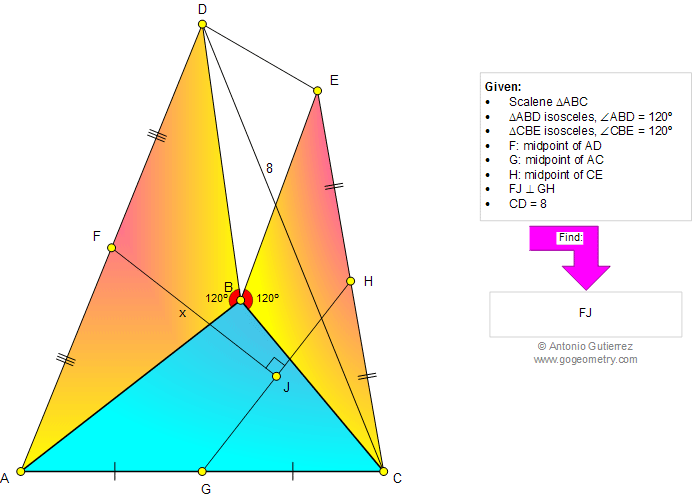# Geometry Problem 1000. Scalene Triangle, Isosceles, Angle, 120 Degree, Midpoint, Distance, Equilateral, Congruence, Perpendicular, Metric Relations. Level: School, College.

< PREVIOUS PROBLEM  |  NEXT PROBLEM >

 The figure below shows a triangle ABC, with triangle isosceles ABD (angle ABD = 120 degree) and triangle isosceles CBE (angle CBE = 120 degree). F, G, and H are midpoints of AD, AC, and CE, respectively. FJ is perpendicular to GH. If CG = 8 find FJ.Home | SearchGeometry | Problems | All Problems | Open Problems | Visual Index | 10 Problems | Problems Art Gallery Art | 991-1000 | Triangles | Isosceles | Equilateral Triangle | Midpoint | 120 degree | Congruence | Perpendicular lines | by Antonio Gutierrez